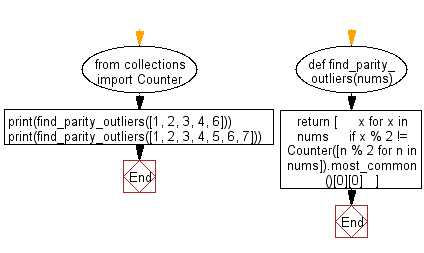﻿ Python: Find the items that are parity outliers in a given list - w3resource# Python: Find the items that are parity outliers in a given list

## Python List: Exercise - 236 with Solution

Write a Python program to find the items that are parity outliers in a given list.

• Use collections.Counter with a list comprehension to count even and odd values in the list.
• Use collections.Counter.most_common() to get the most common parity.
• Use a list comprehension to find all elements that do not match the most common parity.

Sample Solution:

Python Code:

``````from collections import Counter
def find_parity_outliers(nums):
return [
x for x in nums
if x % 2 != Counter([n % 2 for n in nums]).most_common()
]
print(find_parity_outliers([1, 2, 3, 4, 6]))
print(find_parity_outliers([1, 2, 3, 4, 5, 6, 7]))
```
```

Sample Output:

```[1, 3]
[2, 4, 6]
```

Flowchart:## Visualize Python code execution:

The following tool visualize what the computer is doing step-by-step as it executes the said program:

Python Code Editor:

Have another way to solve this solution? Contribute your code (and comments) through Disqus.

What is the difficulty level of this exercise?

Test your Programming skills with w3resource's quiz.

﻿

## Python: Tips of the Day

Invokes the provided function after ms milliseconds:

Example:

```from time import sleep

def tips_delay(fn, ms, *args):
sleep(ms / 1000)
return fn(*args)
print(tips_delay(
lambda x: print(x),
1000,
'w3r'
))
```

Output:

```w3r
None
```